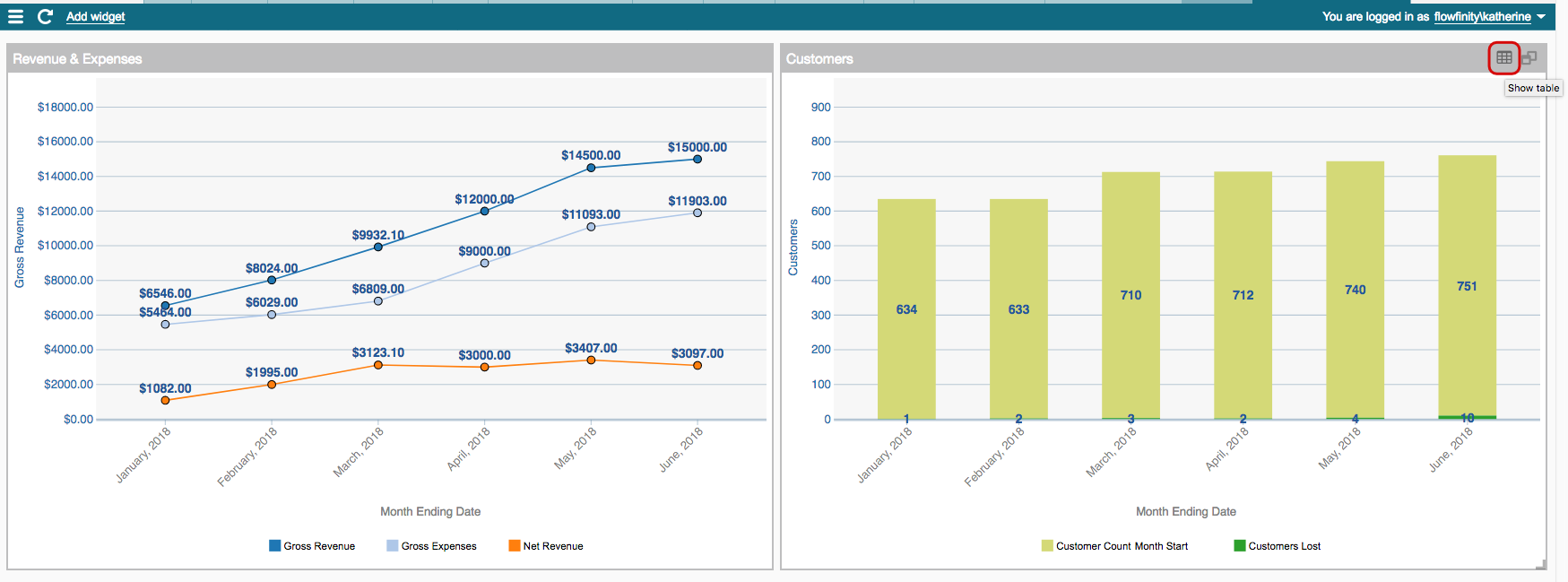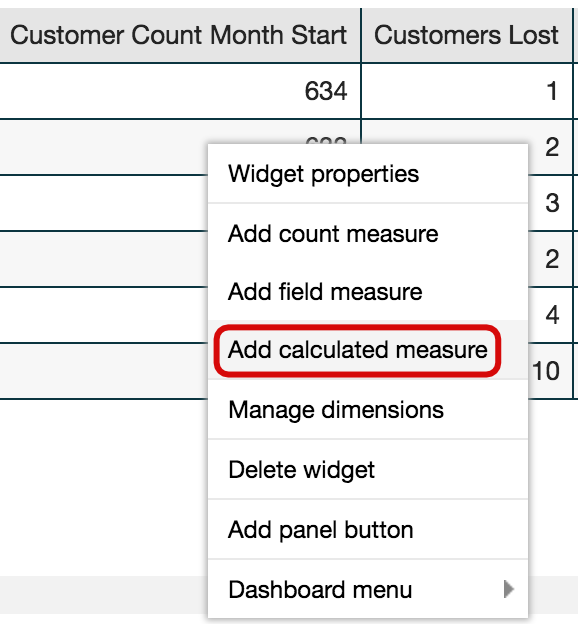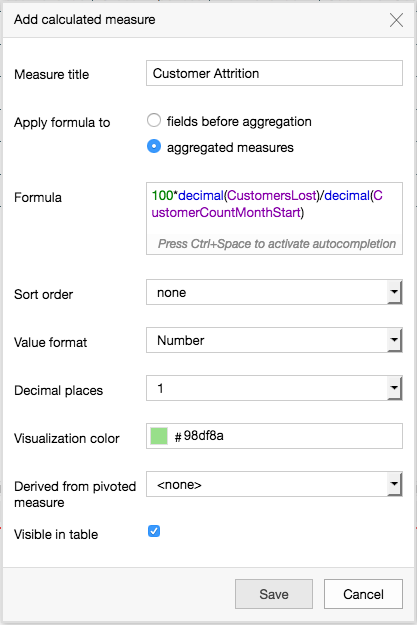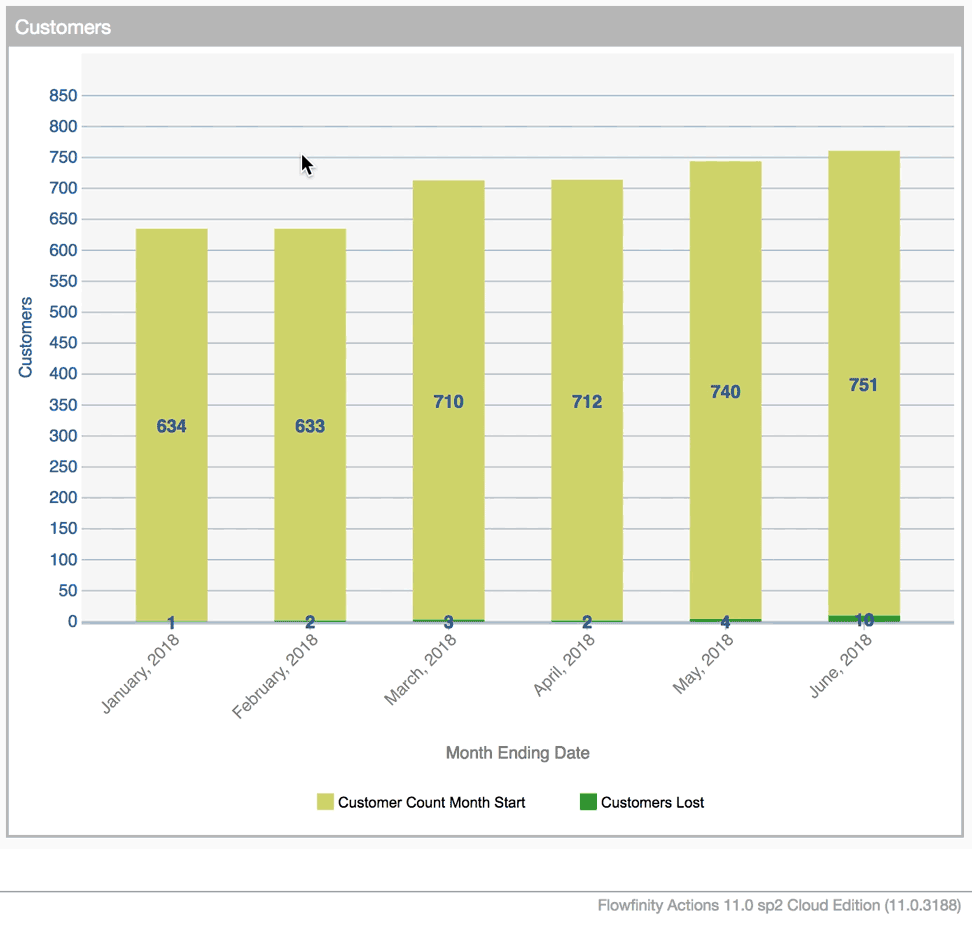﻿ Perform calculations with decimals | Flowfinity Knowledge Base

# Perform calculations with decimals

In apps and dashboards, you can perform numeric calculations and specify whether the results are in integer or decimal format. You can also round the results to a certain number of decimal places.

### Example

This example application tracks monthly revenue, customers and customers lost, and calculates the monthly customer attrition rate.

#### Form Field Calculation

1. Create two numeric fields, "Customer Count Month Start" and "Customers Lost" for the data values we will use in the calculation
2. Create another numeric field called "Customer Attrition Rate" where we will do the calculation
3. Click on "Abc" to the right of field Type and select "Formula Calculation"
4. In the formula section, divide the field "Customers Lost" by "Customer Count Month Start." Press the Control+Spacebar keys to bring up a list of all fields in the app you can select from
5. Check the "Format result as decimal value" checkbox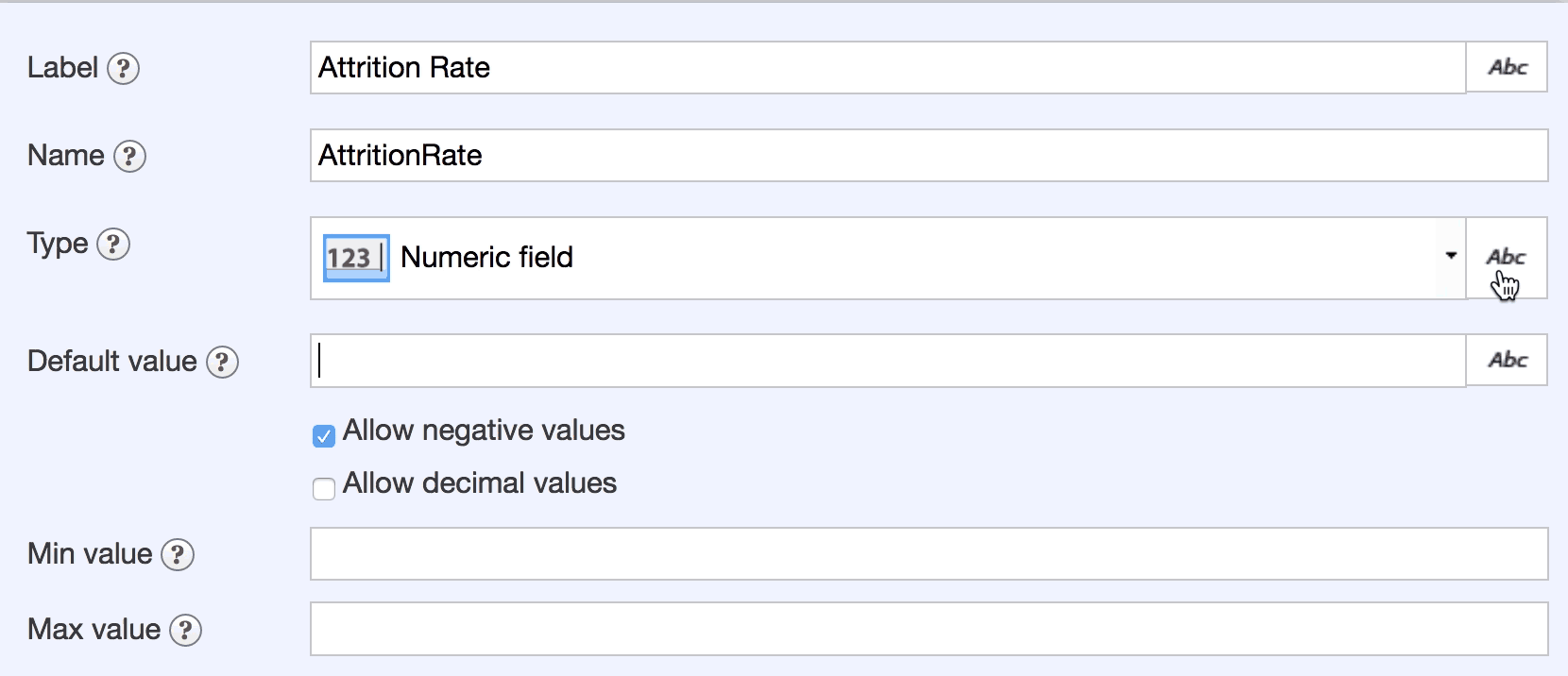6. Next, use Flowfinity's "Decimal" function to force the calculation to treat the two numbers being divided as decimals, rather than integers. This is important to ensure an accurate result because the customer data fields will contain whole numbers which will otherwise be interpreted as integers. The function is written as: Decimal(field)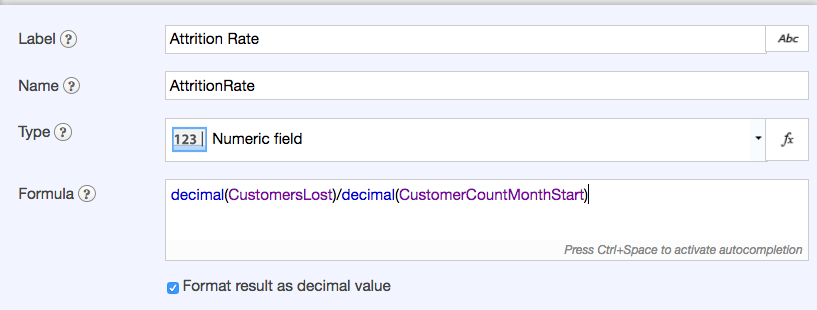7. Multiply by 100 to get percentage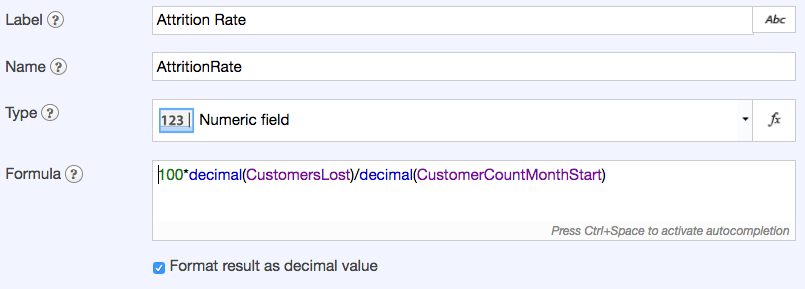8. Use Flowfinity's "Round" function to round the result to one decimal place.
9. The function is written as: Round(field, number of decimal places)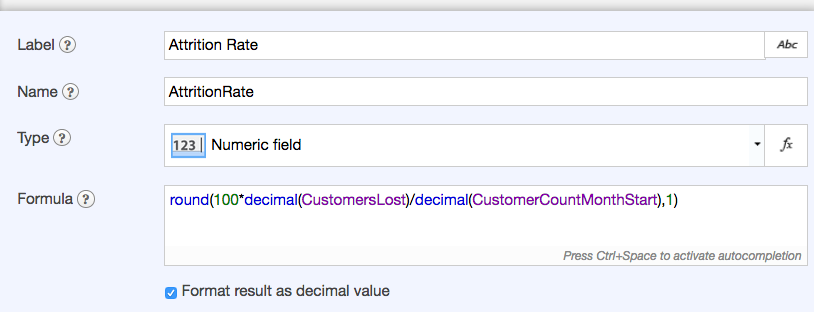Here is the resulting Attrition Rate field shown in a View.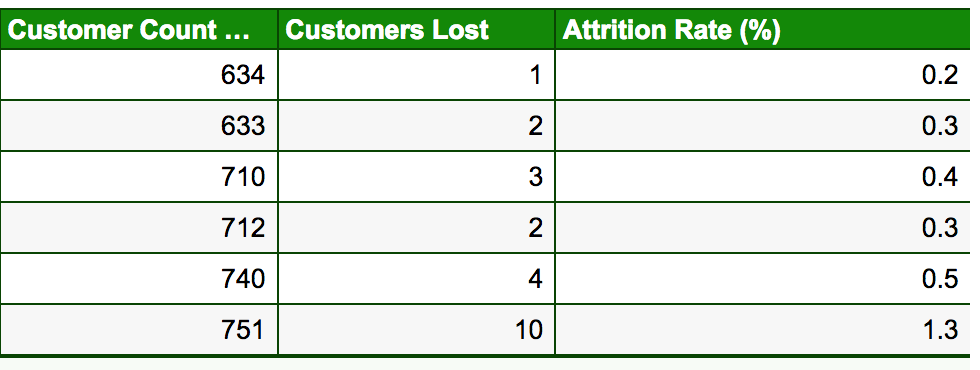#### Dashboard Calculation

You can also perform calculations within dashboards, on data in your database or aggregated data. For example, if you only wish to view the customer attrition rate in a dashboard instead of having a separate field in the application for it, you can perform the calculation directly within the dashboard.

1. Customize the dashboard, from the Configure tab or by clicking the dashboard menu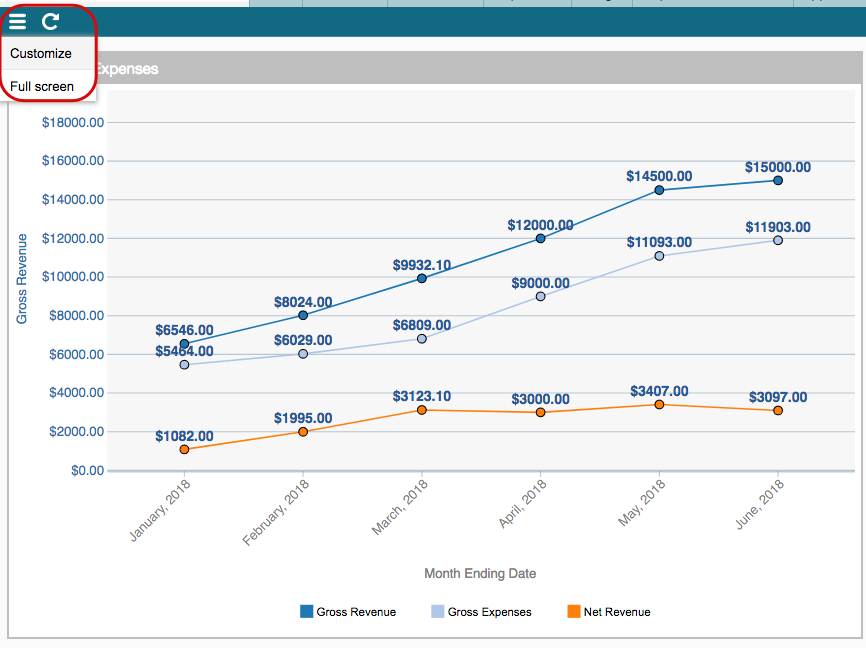2. Click on the table icon to display the data in a table